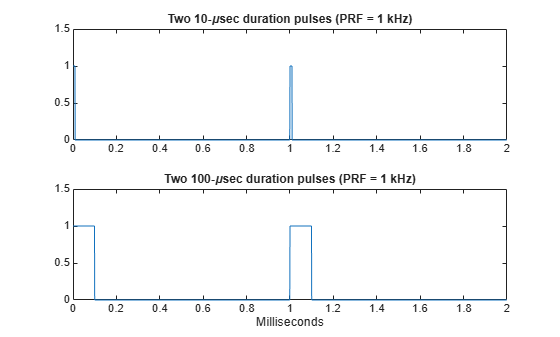## Rectangular Pulse Waveforms

### Definition of Rectangular Pulse Waveform

Define the following function of time:

`$a\left(t\right)=\left\{\begin{array}{ll}1\hfill & 0\le t\le \tau \hfill \\ 0\hfill & \text{otherwise}\hfill \end{array}$`

Assume that a radar transmits a signal of the form:

`$x\left(t\right)=a\left(t\right)\mathrm{sin}\left({\omega }_{c}t\right)$`

where ωc denotes the carrier frequency. Note that a(t) represents an on-off rectangular amplitude modulation of the carrier frequency. After demodulation, the complex envelope of x(t) is the real-valued rectangular pulse a(t) of duration τ seconds.

### How to Create Rectangular Pulse Waveforms

To create a rectangular pulse waveform, use `phased.RectangularWaveform`. You can customize certain characteristics of the waveform, including:

• Sampling rate

• Pulse duration

• Pulse repetition frequency

• Number of samples or pulses in each vector that represents the waveform

### Rectangular Waveform Plot

This example shows how to create a rectangular pulse waveform variable using `phased.RectangularWaveform` . The example also plots the pulse and finds the bandwidth of the pulse.

Construct a rectangular pulse waveform with a duration of 50 μs, a sample rate of 1 MHz, and a pulse repetition frequency (PRF) of 10 kHz.

```waveform = phased.RectangularWaveform('SampleRate',1e6,... 'PulseWidth',50e-6,'PRF',10e3);```

Plot a single rectangular pulse by calling `plot` directly on the rectangular waveform variable. `plot` is a method of `phased.RectangularWaveform`. This method produces an annotated graph of your pulse waveform.

`plot(waveform)`Find the bandwidth of the rectangular pulse.

`bw = bandwidth(waveform)`
```bw = 20000 ```

The bandwidth, `bw`, of a rectangular pulse in hertz is approximately the reciprocal of the pulse duration `1/sRect.PulseWidth`.

### Pulses of Rectangular Waveform

This example shows how to create rectangular pulse waveform signals having different durations. The example plots two pulses of each waveform.

Note: This example runs only in R2016b or later. If you are using an earlier release, replace each call to the function with the equivalent `step` syntax. For example, replace `myObject(x)` with `step(myObject,x)`.

Create a rectangular pulse with a duration of 100 μs and a PRF of 1 kHz. Set the number of pulses in the output equal to two.

```waveform = phased.RectangularWaveform('PulseWidth',100e-6,... 'PRF',1e3,'OutputFormat','Pulses','NumPulses',2);```

Make a copy of your rectangular pulse and change the pulse width in your original waveform to 10 μs.

```waveform2 = clone(waveform); waveform.PulseWidth = 10e-6;```

`sRect` and `sRect1` now specify different rectangular pulses because you changed the pulse width of `waveform`.

Execute the System objects to return two pulses of your rectangular pulse waveforms.

```y = waveform(); y2 = waveform2();```

Plot the real part of the waveforms.

```totaldur = 2*1/waveform.PRF; totnumsamp = totaldur*waveform.SampleRate; t = unigrid(0,1/waveform.SampleRate,totaldur,'[)'); subplot(2,1,1) plot(t.*1000,real(y)) axis([0 totaldur*1e3 0 1.5]) title('Two 10-\musec duration pulses (PRF = 1 kHz)') set(gca,'XTick',0:0.2:totaldur*1e3) subplot(2,1,2) plot(t.*1000,real(y2)) axis([0 totaldur*1e3 0 1.5]) xlabel('Milliseconds') title('Two 100-\musec duration pulses (PRF = 1 kHz)') set(gca,'XTick',0:0.2:totaldur*1e3)```